Introduction
After a study in electrical engineering I joined CDC (Control Data Corporation) in 1968 to install and maintain
mainframes at scientific data centers in the Netherlands
At the decline of CDC in the nineties I went back to college to become a math teacher until retirement in 2009.
My hobby is programming for which I use Delphi.
I am pleased to share the results of my work at this place.

Educational projects
The purpose of software is time saving but also it must stimulate curiosity and experimentation.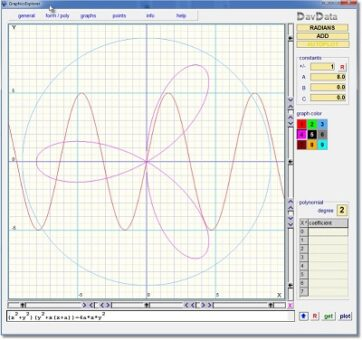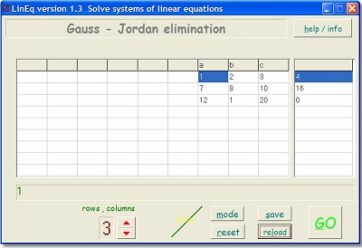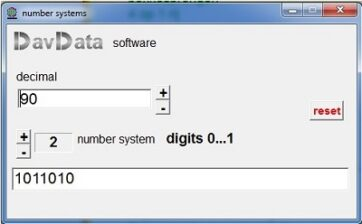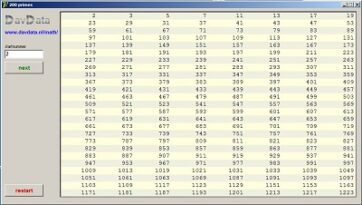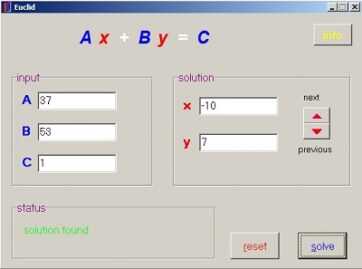Equations of this type originate from situations like:
Children of a deceased collector of paintings execute his last will:

Bernice : 50% of the remaining paintings are yours, grant 1 remaining painting to a museum
Abel : 30% of the paintings are yours. Grant 2 of the remaining paintings to a museum.
Cliff : the remaining pictures are yours

Question: how many paintings are required minimally to make such a partition possible?
Say there are P paintings so at the end Cliff receives C=0.3(0.5P-1)-1 paintings.
This makes the equation 3P-20C=26
a solution is P=22, C=2.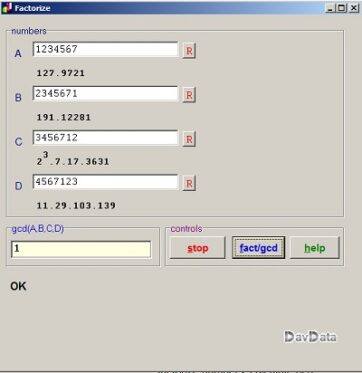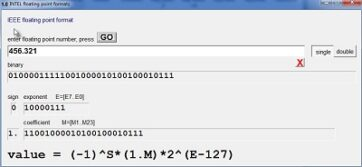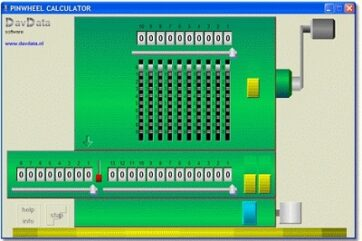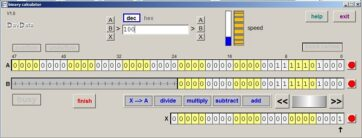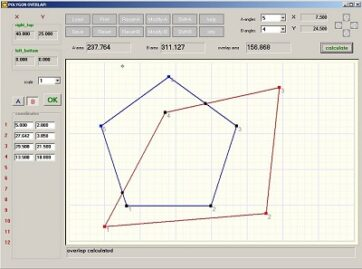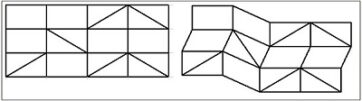Graphics-Explorer
Is a function and equation plotter.
It also supports inverse-, parametric- and implicit functions.

Constants a,b,c are changeable by a mouseclick,
Example: type y = a*sin(b*x+c).
Changing the constants a, b, c shows amplitude, period and phase.
Also Graphic-Explorer calculates polynomials and exponential
equations given a set of points.
Following articles explain algorithms used:

Solve systems of linear equations

Select STEP mode to observe Gauss/Jordan elimination step by step.
Systems of 2x2 to 9x9 equations may be solved.

Number system conversion
This program converts decimal numbers to 2..16 number systems.

Prime numbers generator

Solve equation Ax+By=C
This is a Diophantic equation.
A,B,C,x,y are integers.

Factors are considered the building blocks of numbers.
This program factorizes numbers and calculates
the Greatest Common Divisor (GCD)

Floating point number investigation

For those who are curious what the IEEE format looks like.

DC networks calculator

This program calculates voltages and currents in networks
of voltage sources (batteries) and resistors.
It uses the Kirchhoff laws to build a system of linear equations
which are solved by Gauss elimination.

These machines were used until the 70's.
I got one from my father but my children destroyed it while playing.
So, I decided to write a simulator.
The pinwheel calculator allows for addition, subtraction,
multiplication and division of numbers
almost identical to the pencil and paper method.

similar to the pinwheel calculator above
but bitwise operations.
Carries may be blocked so calculation
is the same as used in checksum generation.

Polygon overlap calculator
This program calculates the overlapping area of two polygons.

Method: divide polygons in triangles and use Heron's formula
to calculate the area.

A large Dutch newspaper had a weekly article called "unclouded" , showing pictures of cities taken from an airplane.
Problem was finding a picture which best matched the area.
People asked me to write a program for this service.
Above is the result.

Stable bookshelves

Bookshelves of horizontal and vertical boards need diagonal
connections to be stable.
Question is: what is the minimal required number of such braces
and where to put them.
This project is an application of graph theory.
Rigid frames correspond to connected bipartite graphs.